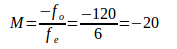# Astronomical telescopes – problems and solutions

1.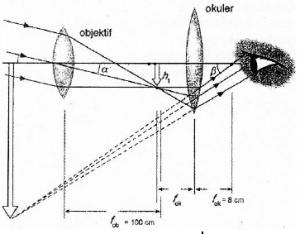Based on the graph above, determine the telescope magnification when the viewing eye is relaxed.

Known :

The lens closest to the object is called the objective lens (focal length fo) and forms a real image. The second lens called the eyepiece (focal length fe) acts as a magnifier.

The focal length of the objective lens (fo) = 100 cm

The focal length of the eyepiece lens (fe) = 8 cm

Wanted : Telescope magnification when eye is relaxed

Solution :

M = -fo / fe

M = -100 cm / 8 cm

M = -12.5 X

[irp]

2.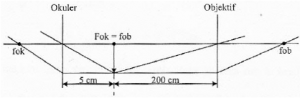Based on the figure above, what is the telescope magnification when the eye is relaxed?

Known :

The focal length of the objective lens (fo) = 200 cm

The focal length of the eyepiece lens (fe) = 5 cm

Wanted : Total magnification

Solution :

When eye is relaxed, the telescope magnification :

M = -fo / fe

M = -200 cm / 5 cm

M = -40 X

[irp]

3.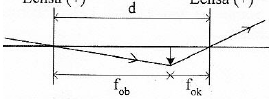Based on above figure, if the distance between the eyepiece lens and the objective lens (d) = 11 times the focal length of the eyepiece lens, then what is the total magnification when the eye is relaxed.

Known :

The distance between the eyepiece lens and the objective lens = the length of telescope = 11 fe

The focal length of the eyepiece lens (fe) = fe

The focal length of the objective lens (fo) = the length of a telescope – the focal length of the eyepiece lens = 11 fe – fe = 10 fe

Wanted: The telescope magnification

Solution :

M = -fo / fe

M = -10 fe / fe

M = -10 X

[irp]

4. What is the total magnification of the astronomical telescope?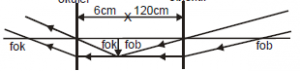Known :

The distance between objective and eyepiece lens (l) = 126 cm

The focal length of the eyepiece lens (fe) = 6 cm

The focal length of the objective lens (fo) = 120 cm

Wanted: The total magnification

Solution :

Based on the above figure, the final image at infinity, so the eye is relaxed.

The total magnification of the astronomical telescope :